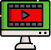VideosNotes

### Arithmetic Of Finance - 1

Arithmetic Of Finance
Arithmetic of Finance means sequence of numbers in which the difference between any two succeeding numbers in the sequence is always the same. The arithmetic mean is the simple average, or sum of a series of numbers divided by the count of that series of numbers. In the world of finance, the arithmetic mean is not usually an appropriate method for calculating an average, especially when a single outlier can skew the mean by a large amount.
Views: N/A
Video Duration: NaN:NaN (Part 1 of 2)
Date Uploaded: March 02, 2021
Grade Level: SS 3
Share this with your friends!
Continue the Series...